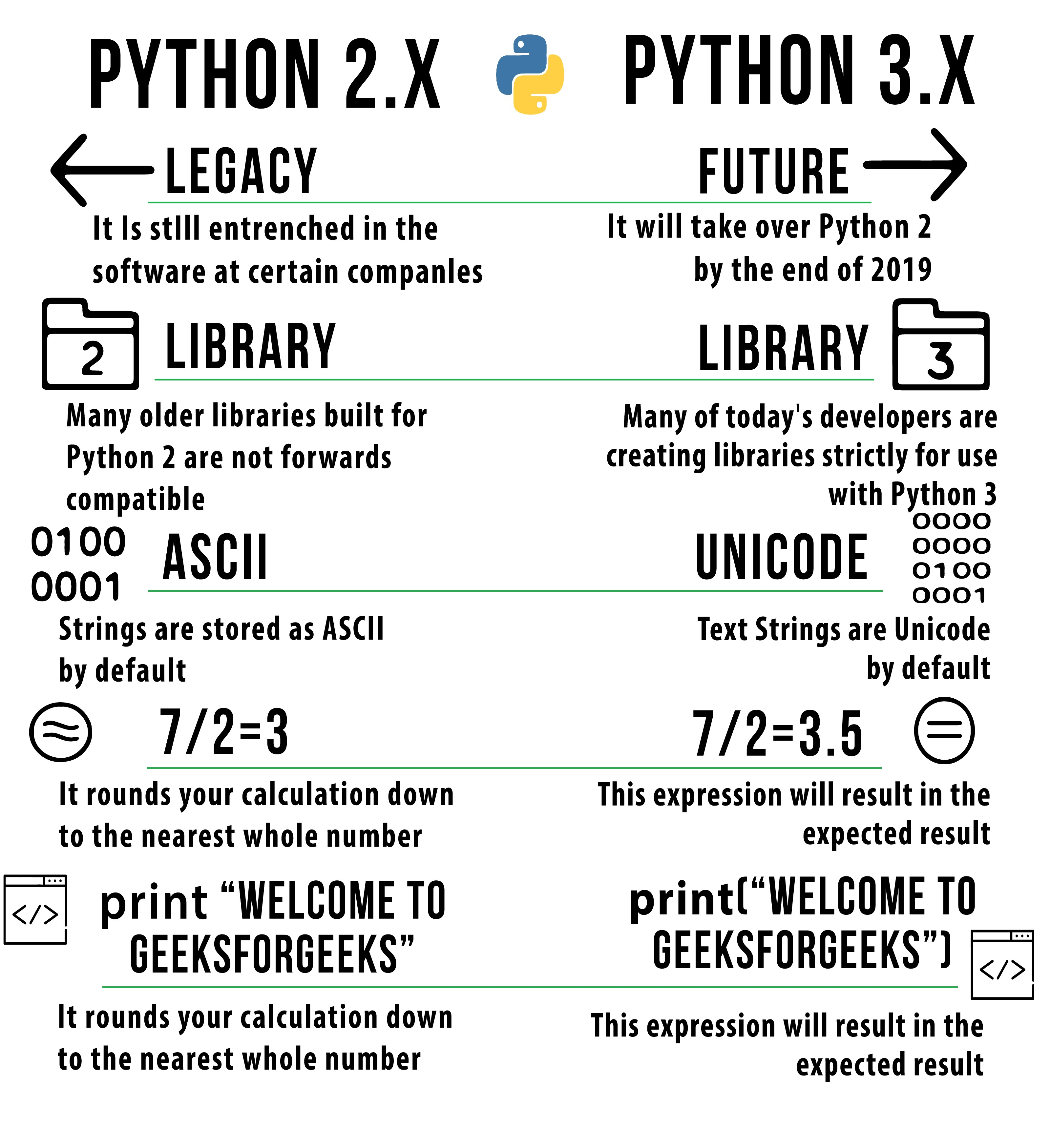# Python2 vs Python3 | Syntax and performance Comparison

Python 2.x has been the most popular version for over a decade and a half. But now more and more people are switching to Python 3.x. Python3 is a lot better than Python2 and comes with many additional features. Also, Python 2.x is becoming obsolete this year. So, it is now recommended to start using Python 3.x from now-onwards.

Still in dilemma?
Ever wondered what separates both of them? Let’s find this thing out below.

First of all, let us go through this quick comparison through this image, which will give you a fair idea on what to expect.Print Statement

Python 2.7: Extra pair of parenthesis is not mandatory in this.

 `print` `'Hello and welcome to GeeksForGeeks'`

Python 3.x: Extra pair of parenthesis is mandatory.

 `print` `(``'Hello and welcome to GeeksForGeeks'``) `

Integer Division

Python 2.7:
The return type of a division (/) operation depends on its operands. If both operands are of type int, floor division is performed and an int is returned. If either operand is a float, a classic division is performed and a float is returned. The // operator is also provided for doing floor division no matter what the operands are.

 `print` `5` `/` `2` `print` `-``5``/``/``2` ` `  `# Output: ` `# 2 ` `# -3 `

Python 3.x:
Division (/) always returns a float. To do floor division and get an integer result (discarding any fractional result) you need to use // operator.

 `print` `(``-``5` `/` `2``) ` `print` `(``5``/``/``2``) ` ` `  `# Output: ` `# -2.5 ` `# 2 `

Input Function

Python 2.7:
When you use input() function, Python automatically converts the data type based on your input.

 `val1 ``=` `input``(``"Enter any number: "``) ` `val2 ``=` `input``(``"Enter any string: "``) ` ` `  `type``(val1) ` `type``(val2) `

`raw_input `gets the input as text (i.e. the characters that are typed), but it makes no attempt to translate them to anything else; i.e. it always returns a string.

 `  `  `val1 ``=` `raw_input``(``"Enter any number: "``) ` `val2 ``=` `raw_input``(``"Enter any string: "``) ` ` `  `type``(val1) ` `type``(val2) `

Python 3.x
In Python3, the input function acts like raw_input from Python 2.7 and it always returns string type.

 `val1 ``=` `input``(``"Enter any number: "``) ` `val2 ``=` `input``(``"Enter any string: "``) ` ` `  `type``(val1) ` `type``(val2) ` `# In order to fix this you need to apply  ` `# float() function when user is prompted for input. `

Round Function

Python 2.7: The output always results in a floating point number.

 `print``(``round``(``69.9``))   ` `print``(``round``(``69.4``)) ` ` `  `# Output:  ` `# 70.0 ` `# 69.0 `

Python 3.x: The return results in n digit precision.

 `print``(``round``(``69.9``))   ` `print``(``round``(``69.4``)) ` ` `  `# Output: ` `# 70 ` `# 69 `

List Comprehensions

Python 2.7: Refer to the example below, how global variable changes.

 `num ``=` `7` `print` `(num) ` ` `  `mylist ``=` `[num ``for` `num ``in` `range``(``100``)] ` `print` `(num) ` ` `  `# Output: ` `# 7 ` `# 99 `

Python 3.x: There is no namespace leak now. This is quite fixed now.

 `num ``=` `7` `print` `(num) ` ` `  `mylist ``=` `[num ``for` `num ``in` `range``(``100``)] ` `print` `(num) ` ` `  `# Output:  ` `# 7 ` `# 7 `

Range Function

Python 2.7 :
It has both `range `and `xrange `function. When you need to iterate one object at a time, use xrange and when you need an actual list, use range function. xrange is generally faster & saves memory.

 `%` `timeit [i ``for` `i ``in` `range``(``1000``)]   ` `%` `timeit [i ``for` `i ``in` `xrange``(``1000``)] `

Python 3.x :
Here range does what xrange does in Python 2.7. xrange doesn’t work in Python 3.x.

 `%` `timeit [i ``for` `i ``in` `range``(``1000``)]   ` `%` `timeit [i ``for` `i ``in` `xrange``(``1000``)] `

Exception Handling

Python 2.7 : This has a different syntax than Python 3.x.

 `try``: ` `    ``YoYo ` `except` `NameError, error: ` `    ``print` `error, ``"YOU HAVE REACHED FOR AN ERROR"` ` `  `try``: ` `    ``YoYo ` `except` `NameError as error: ` `    ``print` `error, ``"YOU HAVE REACHED AN ERROR, YET AGAIN !"`

Python 3.x: ‘As’ keyword is needed to be included in this.

 `try``: ` `    ``YoYo ` `except` `NameError as error: ` `    ``print` `(error, ``"THE ERROR HAS ARRIVED !"``) `

List Comprehensions

Python 2.7: Lesser parenthesis than Python 3.x.

 `[item ``for` `item ``in` `1``, ``2``, ``3``, ``4``, ``5``] ` `[``1``, ``2``, ``3``, ``4``, ``5``] `

Python 3.x: Extra pair of parenthesis is needed here.

 `[item ``for` `item ``in` `(``1``, ``2``, ``3``, ``4``, ``5``)] ` `[``1``, ``2``, ``3``, ``4``, ``5``] `

next() function and .next() method

Python 2.7: Both next() and .next() are used here.

 `generator ``=` `(letter ``for` `letter ``in` `'abcdefg'``) ` `next``(generator) ` `generator.``next``() `

Python 3.x: Only next() is used here. Using .next() shows an AttributeError.

 `generator ``=` `(letter ``for` `letter ``in` `'abcdefg'``) ` `next``(generator) `

ASCII, Unicode and Byte types

Python 2.7: It has ASCII string type, a separate unicode type, but there is no byte type.

 `type``(``unicode``(``'a'``)) ` `type``(u``'a'``) ` `type``(b``'a'``) `

Python 3.x: We have unicode strings, and byte type.

 `type``(``unicode``(``'a'``)) ` `# This returns an error `

Note: List of Methods & Functions that don’t return list anymore in Python 3.x.

```In Python2.x -

zip()
map()
filter()
dictionary’s .keys() method
dictionary’s .values() method
dictionary’s .items() method
```

My Personal Notes arrow_drop_upCheck out this Author's contributed articles.

If you like GeeksforGeeks and would like to contribute, you can also write an article using contribute.geeksforgeeks.org or mail your article to contribute@geeksforgeeks.org. See your article appearing on the GeeksforGeeks main page and help other Geeks.

Please Improve this article if you find anything incorrect by clicking on the "Improve Article" button below.

Improved By : Code_r, nidhi_biet

Article Tags :

4

Please write to us at contribute@geeksforgeeks.org to report any issue with the above content.